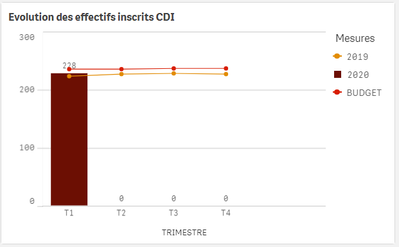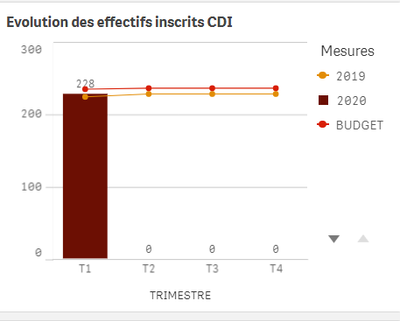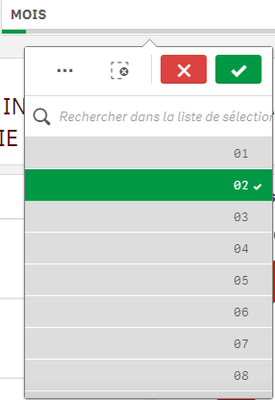# New to Qlik Sense

If you’re new to Qlik Sense, start with this Discussion Board and get up-to-speed quickly.

Announcements
An issue has been identified on Qlik Cloud hub, please visit our Status Update Page for details: GET THE LATEST
cancel
Showing results for
Did you mean:Creator

## Set expression to exclude month selection

Hello everyone,I have this bar chart which express 3 measures over the course of 4 quarter 'T1 = Quarter 1 and so on'.

For the measure year 2019, my set of expression is the following one:

if (TRIMESTRE = 'T1' ,SUM({<CAT_CONTRAT = {'CDI'}, Annee = {'\$(=vPreviousYear)'},
Mois = {'03'} , TRIMESTRE = {'T1'} >}EFF_FINMOIS),

if (TRIMESTRE = 'T2',SUM({<CAT_CONTRAT = {'CDI'}, Annee = {'\$(=vPreviousYear)'},
Mois = {'06'} , TRIMESTRE = {'T2'}>}EFF_FINMOIS),

if (TRIMESTRE = 'T3' ,SUM({<CAT_CONTRAT = {'CDI'}, Annee = {'\$(=vPreviousYear)'},
Mois = {'09'}, TRIMESTRE = {'T3'}>}EFF_FINMOIS),

if (TRIMESTRE = 'T4' ,SUM({<CAT_CONTRAT = {'CDI'}, Annee = {'\$(=vPreviousYear)'},
Mois = {'12'}, TRIMESTRE = {'T4'}>}EFF_FINMOIS),))))

But the issue is that the result doesn't display value for quarter 2, 3 and 4 because i have a filter box with month = '02'.

So the result is:My variable is vPreviousYear  =year(vToday)-1

My question is how is it possible to allow my set anbalysis to work without the selection month = 2 impact it ?

Thank you

Labels (2)

• ### Set Analysis

7 RepliesMaster III

Try excluding the Month in set analysis

SUM({<CAT_CONTRAT = {'CDI'}, Annee = {'\$(=vPreviousYear)'},
Mois = {'03'} , TRIMESTRE = {'T1'},Month= >}EFF_FINMOIS)Creator
Author

If i add Mois=  ((Mois = Month field)

SUM({<CAT_CONTRAT = {'CDI'}, Annee = {'\$(=vPreviousYear)'},
Mois = {'03'} , TRIMESTRE = {'T1'},Month= >}EFF_FINMOIS)

So the set analysis exclude the Month field (= Mois in french) basically the chart doesn't take into account the previous month i put in set analysis which is Mois = 03 (Month = 03 so march)Master III

Can you share sample app with expected outputCreator
Author

Here is the sample !

I would like my chart to display the data on quarter 1 2 3 and (in the chart T1 = Quarter 1) previous year.

But when you select the filter Mois (=Month) = '02' (february) the chart display only data from that period of time  .

Basically i want to display data from 2019 (Measure = 2019) ( The filter applied is CAT-SALARIE = 'AR')Like this (in the sample there is no the budget measure)

But while selecting the month value '02'I get this (not what i want)MVP

``````use below expression and same you can apply for Budget
hightlights : if(only({<Mois>}TRIMESTRE) = 'T1'

if (only({<Mois>}TRIMESTRE) = 'T1' ,SUM({<CAT_CONTRAT = {'CDI'}, Annee = {'\$(=vPreviousYear)'},
Mois ={'03'} >}EFF_FINMOIS),

if (only({<Mois>}TRIMESTRE) = 'T2',SUM({<CAT_CONTRAT = {'CDI'}, Annee = {'\$(=vPreviousYear)'},
Mois = {'\$(=if(GetSelectedCount(Mois) > 0, only({<Mois = {'\$(vMois_CDI_T2_N-1)'}>}Mois)))'} >}EFF_FINMOIS),

if (only({<Mois>}TRIMESTRE) = 'T3' ,SUM({<CAT_CONTRAT = {'CDI'}, Annee = {'\$(=vPreviousYear)'},
Mois = {'09'} , TRIMESTRE = {'T3'}>}EFF_FINMOIS),

if (only({<Mois>}TRIMESTRE) = 'T4' ,SUM({<CAT_CONTRAT = {'CDI'}, Annee = {'\$(=vPreviousYear)'},
Mois = {'12'} , TRIMESTRE = {'T4'}>}EFF_FINMOIS)))))``````Creator
Author

Thanks A LOT !

I now understand my mistake.

I had to exclude the month selection into each TRIMESTER FIELD.

Thank you allMVP